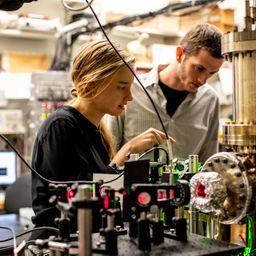## a. 2.24 e Vb. 5.55 \times 10^{-7} e Vc. 5.41 \times 10^{14} \mathrm{Hz}

Quantum Physics

Atomic Physics

### Discussion

You must be signed in to discuss.
##### Top Physics 103 Educators##### Marshall S.

University of Washington##### Jared E.

University of Winnipeg### Video Transcript

Okay, So in this problem, um, we know that light has a wavelength of 3 350 nanometers. So let's go ahead and write that out. 3 50 10 times, 10 to the minus nine nano meters. And, um, thehe Let Tron Czar omitted from this potassium surface with 1.31 e v. So K equals 1.1. You, me, 1.131 TV. My mind you. It's full of these numbers. And I'm trying to remember them as I tap over, um, and then we want to find the work function of potassium. So what we want to do is no acknowledge that K e is equal to, um so it's equal the energy of the photons. That's HC over Lambda, minus the work function. So the work function is equal to age. See, over Lambda Times, my s K e e, and plugging all of this into a calculator. Pause the video while I do that. I got 2.23 evey and next we want to get the cutoff wavelength and the cut off frequency the coat so that kind of would be if you reject the electrons that they don't have any extra energy. So, um, you get the cut off. I'm gonna go ahead. Label this a So for being of the cut off by setting kinetic energy equal to zero. So you get ht over Lambda is fi. And so then you consult for Lambda equals each see divided by five. So if I go ahead and plug that into a calculator, I'm gonna pause the video. I do that. Okay, um, I calculated that, and I got 555 nanometers for the cutoff wavelength. And then the corresponding frequency is gonna be e over H um And then he is equal to HC Overland Us. I guess that frequency is gonna be C over lambda. So calculating that I got 5.40 times 10 of the 14 hurts.University of Washington

#### Topics

Quantum Physics

Atomic Physics

##### Top Physics 103 Educators##### Marshall S.

University of Washington##### Jared E.

University of Winnipeg